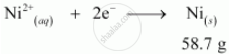Share

# A solution of Ni(NO3)2 is electrolysed between platinum electrodes using a current of 5 amperes for 20 minutes. What mass of Ni is deposited at the cathode? - CBSE (Science) Class 12 - Chemistry

#### Question

A solution of Ni(NO3)2 is electrolysed between platinum electrodes using a current of 5 amperes for 20 minutes. What mass of Ni is deposited at the cathode?

#### Solution

Given,

Current = 5A

Time = 20 × 60 = 1200 s

∴ Charge = current × time

= 5 × 1200

= 6000 C

According to the reaction,Nickel deposited by 2 × 96487 C = 58.71 g

Therefore, nickel deposited by 6000 C = (58.71xx6000)/(2xx96487)g

= 1.825 g

Hence, 1.825 g of nickel will be deposited at the cathode.

Is there an error in this question or solution?

#### Video TutorialsVIEW ALL 

Solution A solution of Ni(NO3)2 is electrolysed between platinum electrodes using a current of 5 amperes for 20 minutes. What mass of Ni is deposited at the cathode? Concept: Fuel Cells.
S